# Two workers

One worker needs 40 hours to do a job, and the second would do it in 30 hours. They worked together for several hours, then the second was recalled, and the first completed the job itself in 5 hours. How many hours did they work together, and how much did each of them do?

Correct result:

x =  15 h
a =  0.5
b =  0.5

#### Solution:

$a=\frac{x+5}{40}=\frac{15+5}{40}=\frac{1}{2}=0.5$
$b=\frac{x}{30}=\frac{15}{30}=\frac{1}{2}=0.5$We would be pleased if you find an error in the word problem, spelling mistakes, or inaccuracies and send it to us. Thank you!Tips to related online calculators
Need help calculate sum, simplify or multiply fractions? Try our fraction calculator.
Do you have a linear equation or system of equations and looking for its solution? Or do you have quadratic equation?
Do you want to convert time units like minutes to seconds?

## Next similar math problems:

• Workers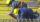Workers A and B will done work together for 15 days. They worked together 13.5 days. Then A worker became ill and worker B finish the job alone for 7.5 days. If every worker was working alone, how many days took whole work for worker A and B?
• Two workersTwo workers should fulfill certain task together for 5 days. If the first worker increased their performance twice and second twice fell, it took them just four days. For how many days would handle the entire task first worker himself?
• Tractors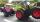Two tractors plow the field in 4 hours together. If the first tractor plow half of the field and then the second tractor completed the job, it would take 9 hours. How many hours does the field plow for each tractor separately?
• Three workers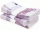The three workers received € 2,850 together for the work done. They divided them according to the time worked so that the first received 20% less than the second and the third € 50 more than the second. How much EUR did each worker receive?
• Working together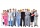Two people will do the work in 12 days. They worked together for 8 days. Then only one worked for 10 days. How many days would each of them do the work if he worked alone?
• Forestry workersIn the forest is employed 56 laborers planting trees in nurseries. For 8 hour work day would end job in 37 days. After 16 days, 9 laborers go forth? How many days are needed to complete planting trees in nurseries by others, if they will work 10 hours a d
• Two workers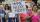The first worker completed the task by himself in 9 hours, the second in 15 hours. After two hours of joint work left first worker to a doctor and the second finished the job himself. How many hours worked second worker himself?
• Two companies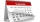The family ordered two companies to modify the garden. The first would be done in 20 days, the second would take 40% less time. How long will they work together if the second company started working when the first worked for 4 days?
• Two diggersTwo diggers should dig a ditch. If each of them worked just one-third of the time that the other digger needs, they'd dig up a 13/18 ditch together. Find the ratio of the performance of this two diggers.
• Two workersThe first worker would himself completed the work in 8 hours, the other in 6 hours. After two hours of joint work left the first worker to a doctor and other completed work himself. How many hours worked the other worker himself?
• Three workersThree workers were rewarded CZK 9200 and the money divided by the work they have done. First worker to get twice than the second, the second three times more than the third. How much money each worker received?
• Boots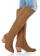Master shoemaker has three apprentices. First do one pair of boots for two days, second for 1 day, third on 1.5 day. If they worked together, for how long it would take made a couple of boots?
• WorkThe first worker would need less than 4 hours to complete the task than the other worker. In fact, both workers worked for two hours together, then the first worker did the remaining work himself. In what proportion should the remuneration of the workers
• Chickens and rabbitsIn the yard were chickens and rabbits. Together they had 27 heads and 86 legs. How many chickens and how many rabbits were in the yard?
• Two workers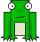Two workers together execute some work in 10 days. The first worker would have done himself in 20 days. How many days would have done himself a second worker?
• Two workers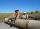Two workers carry will do certain work for 12 days. After 8 days of working was one removed, and then the other finished the job alone in 10 days. For how many days would do this work alone each worker?
• Mason with assistantMason had to complete the work in 10 days. Two and a half days later his assistant arrived. Together they completed the work in next three and a half days. How many days would he need an assistant for the same work?